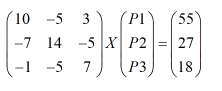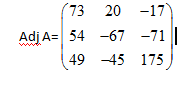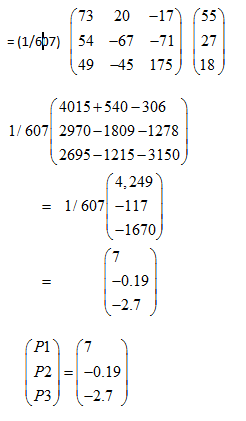# Q3 Use a matrix method to find the equilibrium prices and quantities where the supply and demand functions for Good 1, Good 2 and Good 3 are as Qd1 = 50 − 2P1 + 5P2 − 3P3, Qs1 = 8P1 − 5 Qd2 = 22 + 7P1 − 2P2 + 5P3, Qs2 = 12P2 − 5 Qd3 = 17 + P1 + 5P2 − 3P3, Qs3 = 4P3 − 1

Question
79 views

Q3

Use a matrix method to find the equilibrium prices and quantities where the supply and demand functions
for Good 1, Good 2 and Good 3 are as
Qd1 = 50 − 2P1 + 5P2 − 3P3, Qs1 = 8P1 − 5
Qd2 = 22 + 7P1 − 2P2 + 5P3, Qs2 = 12P2 − 5
Qd3 = 17 + P1 + 5P2 − 3P3, Qs3 = 4P3 − 1

check_circle

Step 1

Step 1:

To find equilibrium, we’ll equate quantity demanded l to quantity supplied.

Qd1=Qs1

50-2P1+5P2-3P3=8P1-5

10P1-5P2+3P1=55                           . . . . . . . .  (1)

Qd2=Qs2

22+7P1-2P2+5P3=12P2-5

-7P1+14P2-5P3=27                           . . . . . . . .  (2)

Qd3=Qs4

17+P1+5P2-3P3=4P3-1

-P1-5P2+7P3=18                                 . . . . . . . .  (3)

Step 2

Now, we’ll write above three equations in a matrix form AY.A is 3x3 matrix having coefficients of P1,P2 and P3, Y is a column matrix of P1,P2,P3 and B is also a column matrix having constant terms.

Step 3

Y=A-1B

A-1= (1/Det A) x (Adj A)

Det A= 10{(14x7)-(-5x5)}-5{(-7x7)-(5x-1)}+3{(-7x5)-(14x-1)}

= 730-270+147

=607Y=A-1BAs price can’t be negative, so we simply ignore minus sign.

Therefore, P1...

### Want to see the full answer?

See Solution

#### Want to see this answer and more?

Solutions are written by subject experts who are available 24/7. Questions are typically answered within 1 hour.*

See Solution
*Response times may vary by subject and question.
Tagged in